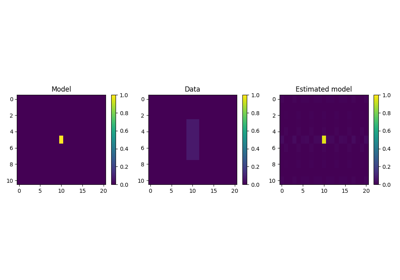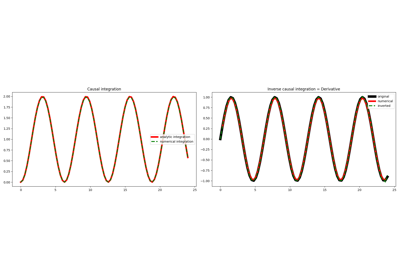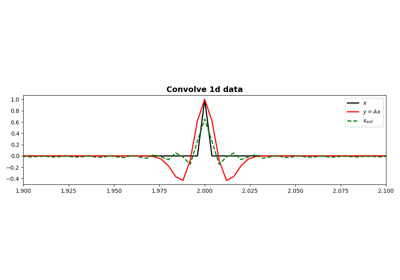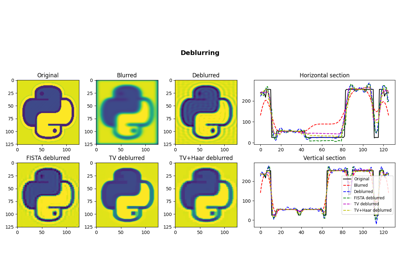# pylops.signalprocessing.ConvolveND¶

class pylops.signalprocessing.ConvolveND(dims, h, offset=None, axes=(-2, -1), method='fft', dtype='float64', name='C')[source]

ND convolution operator.

Apply n-dimensional convolution with a compact filter to model (and data) along the axes of a n-dimensional array.

Parameters: dims : Number of samples for each dimension h : numpy.ndarray nd compact filter to be convolved to input signal offset : tuple, optional Indices of the center of the compact filter axes : int, optional New in version 2.0.0. Axes along which convolution is applied method : str, optional Method used to calculate the convolution (direct or fft). dtype : str, optional Type of elements in input array. name : str, optional New in version 2.0.0. Name of operator (to be used by pylops.utils.describe.describe)

Notes

The ConvolveND operator applies n-dimensional convolution between the input signal $$d(x_1, x_2, ..., x_N)$$ and a compact filter kernel $$h(x_1, x_2, ..., x_N)$$ in forward model. This is a straighforward extension to multiple dimensions of pylops.signalprocessing.Convolve2D operator.

Attributes: shape : tuple Operator shape explicit : bool Operator contains a matrix that can be solved explicitly (True) or not (False)

Methods

 __init__(dims, h[, offset, axes, method, …]) Initialize this LinearOperator. adjoint() Hermitian adjoint. apply_columns(cols) Apply subset of columns of operator cond([uselobpcg]) Condition number of linear operator. conj() Complex conjugate operator div(y[, niter, densesolver]) Solve the linear problem $$\mathbf{y}=\mathbf{A}\mathbf{x}$$. dot(x) Matrix-matrix or matrix-vector multiplication. eigs([neigs, symmetric, niter, uselobpcg]) Most significant eigenvalues of linear operator. matmat(X) Matrix-matrix multiplication. matvec(x) Matrix-vector multiplication. reset_count() Reset counters rmatmat(X) Matrix-matrix multiplication. rmatvec(x) Adjoint matrix-vector multiplication. todense([backend]) Return dense matrix. toimag([forw, adj]) Imag operator toreal([forw, adj]) Real operator tosparse() Return sparse matrix. trace([neval, method, backend]) Trace of linear operator. transpose() Transpose this linear operator.

## Examples using pylops.signalprocessing.ConvolveND¶2D SmoothingCausal IntegrationConvolution05. Image deblurring